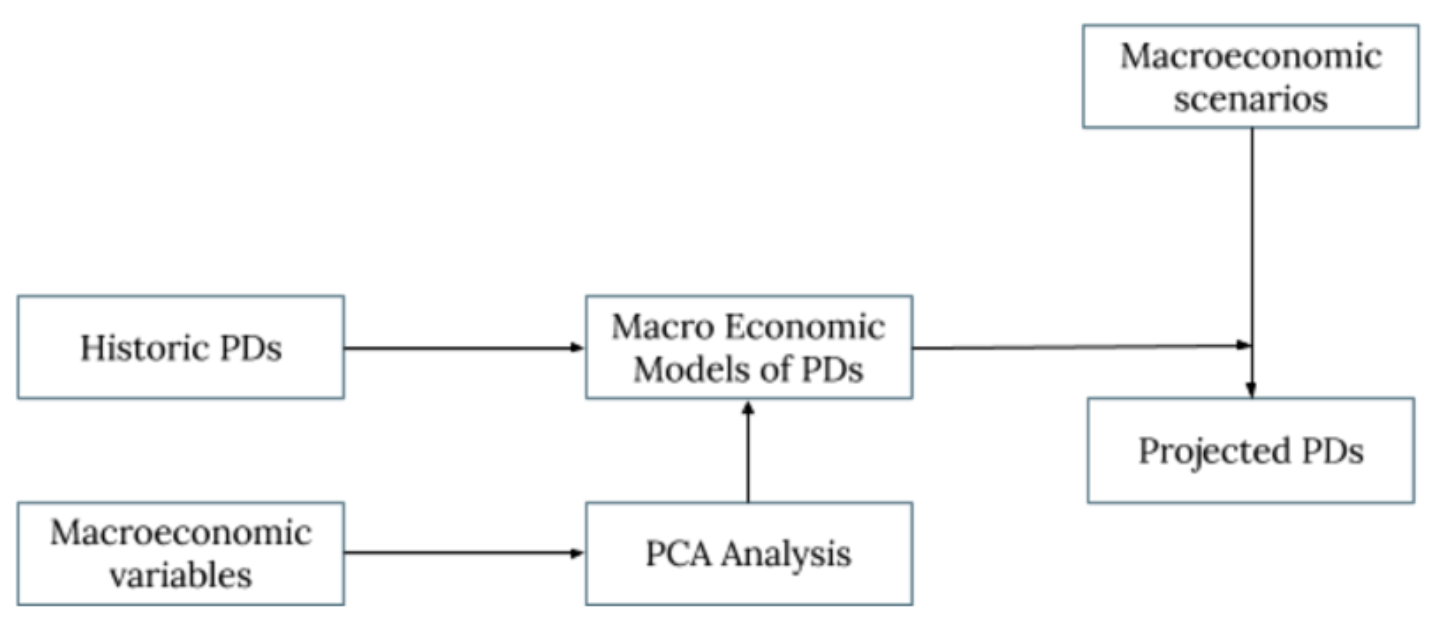# What is Expected Credit Loss (ECL)?

Based on a risk, banks and financial institutes want to take actions in these rapidly growing financial markets and one of most important risk that they are exposed to is credit risk. ECL is used to recognize the impairment losses arising from potential defaults in future.

The most common methodology for estimating ECL is the probability of default approach which takes 3 inputs into consideration that is Exposure At Default (EAD), Probability Of Default (PD) and Loss Given Default (LGD).

PD: It is the likelihood over a specific period (12 months or life time) that the borrower will not be able to pay back a debt.

LGD: Percentage that the financial institute can lose when borrower defaults

EAD: The amount that the borrower has not payed back at the time of default

ECL= PD x LGD x EAD

Where,

• The above equation assumes, PD, EAD and LGD remain constant throughout the year. Constant EAD assumption is especially wrong in cases of repayment (planned or unplanned) and additional drawdowns.
• This is an approximation and more accurate method is given by:Where,

MPDt is marginal probability of default at time t

LGDt is loss given default at time t

EADt is exposure at default at time t

Dft is discounting factor at time t

• If we take a conservative approach, Df can be taken as 1 through that year. This will slightly overestimate the ECL. The ECL becomes:

ECL = PD x LGD x Avg. EAD

• If the facility has monthly payments, the above equation can be written as:
• Where, each term represents MPD, LGD, EAD for each month. As we are projecting PD for one year, the marginal PD for each month will be 1/12 of annual PD. LGD is constant throughout the year. So,

• The Average EAD used in the above equation should be the average expected balance of the facility after adjusting for repayment and drawdowns. If it is a uniform repayment (or close to uniform), the average EAD can be approximated by:

• This is for calculating one year expected loss. For multiple year expected loss marginal PDs and exposures should be extended for all the years through the life time of the exposure
• To measure ECL the first step is to perform PD modelling to get the probability of default. We have written an elaborate blog on the same, the summary is below.

# Macro-economic PD Modelling

We take macroeconomic factors like GDP growth rate, Inflation, crude oil price and Government spending for the prediction of PDs.After testing collinearity we perform log transformation to get normalised data and then standardised the factors to perform further modelling. It requires linking of historic PDs (quarterly) for each risk Transition PD buckets through Principal Component Analysis and Logistic regression.

# Applications of ECL:

Banks and other institutions are working on ECL frameworks for mainly accounting purposes. But ECL has much wider applications including:

1.  Internal monitoring and performance evlautions

2. Creating provision for future losses

3. Financial reporting

4. Risk management of various segments

 ECL methodology explanation:

https://assets.kpmg/content/dam/kpmg/in/pdf/2017/07/Demystifying-Expected-Credit-Loss.pdf

Follow